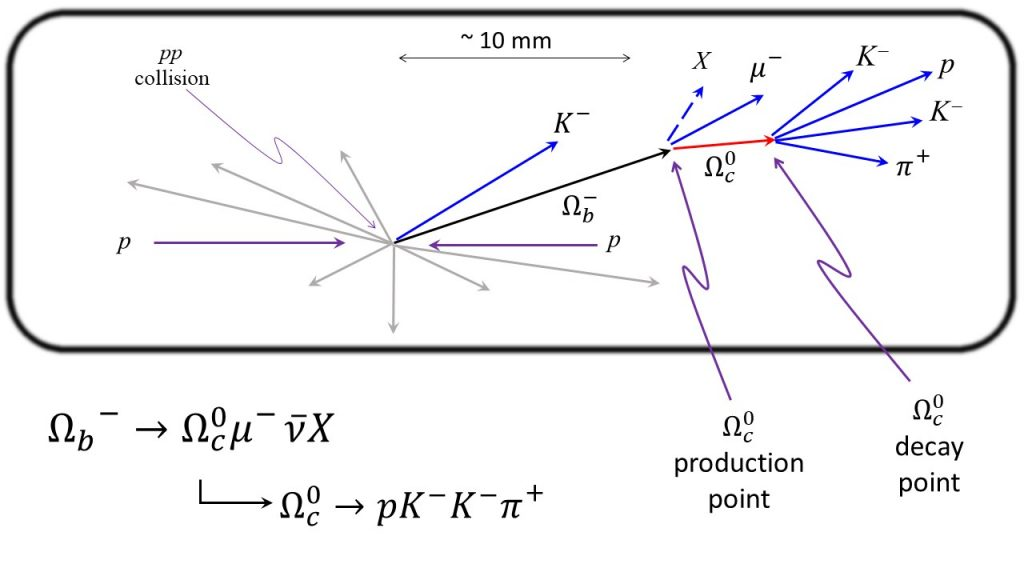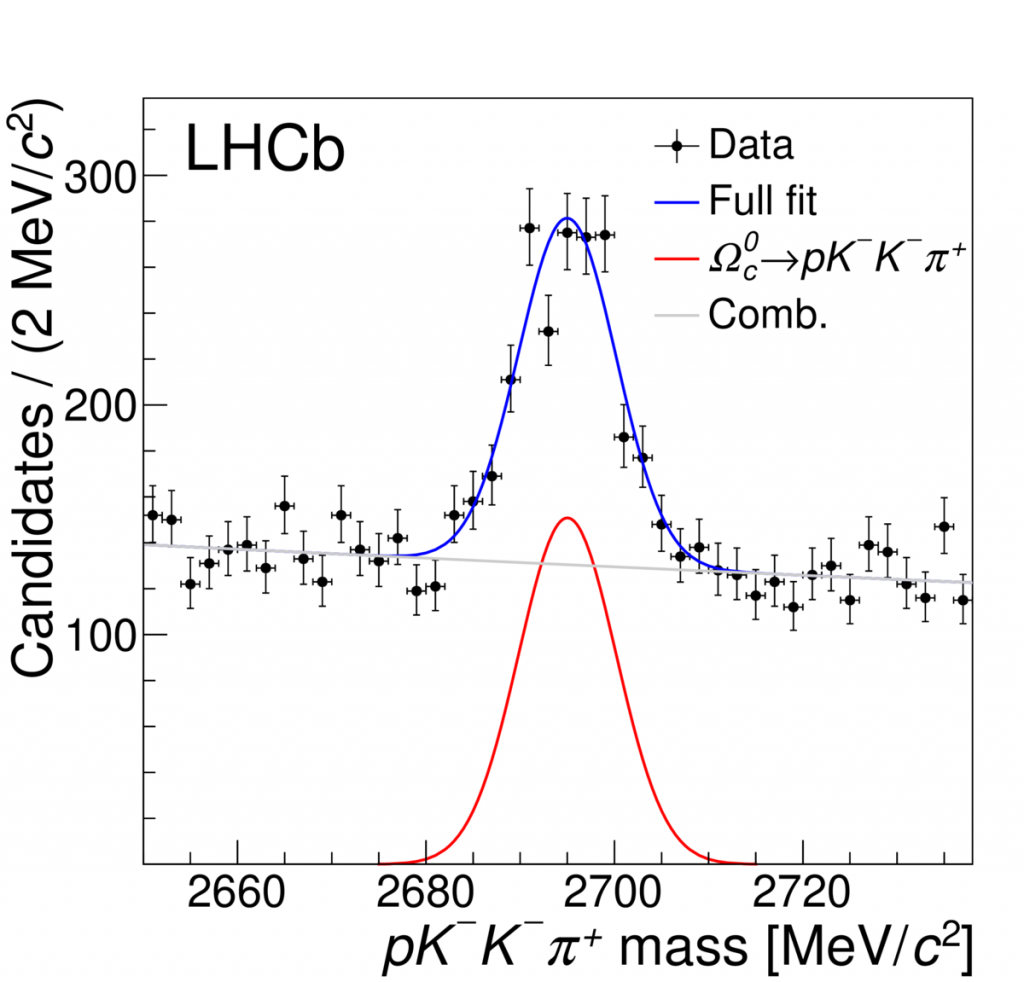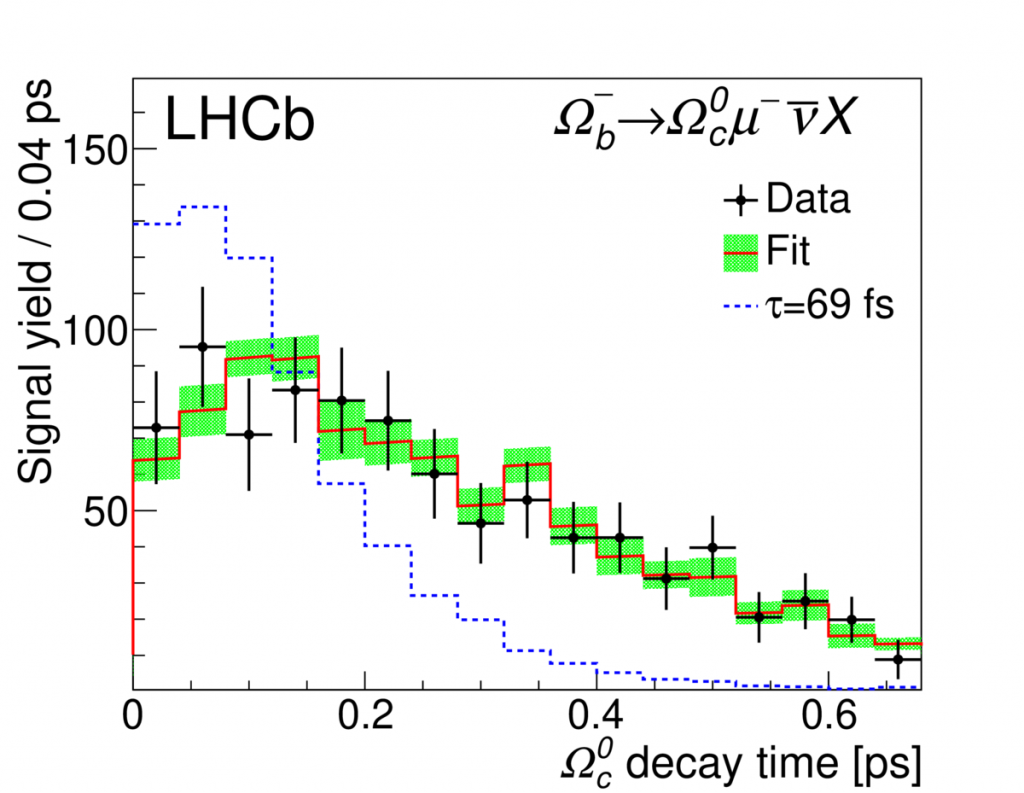# Measurement of the Ωc0 baryon lifetime

One of the basic properties of particles is their lifetime, or, in the case of strong decays, the natural width. The lifetimes of weakly-decaying beauty and charm hadrons can be predicted using the so-called Heavy Quark Expansion (HQE), which is a perturbative expansion of the total decay rate (Γ) in powers of 1/mb, where mb is the b-quark mass. Since the b-quark mass is large, it is expected that the lowest order terms dominate, and the expansion should give predictive power for the beauty total decay rate. Since the beauty hadron lifetimes, τ, are related to the total decay rate through τ = ℏ/Γ this allows for a prediction of τ.

The HQE can also be applied to charm hadrons. However, since the charm-quark mass, mc, is not that large, higher orders terms in the expansion yield larger corrections, and one would expect more terms in the expansion could be significant. Because of this, measuring charm-hadron lifetimes is a sensitive probe to the HQE, particularly the higher-order terms and their associated matrix elements.

The lifetimes of all (weakly-decaying) charm and beauty hadrons discovered so far have been measured. However, the lifetime of the Ωc0 baryon (quark content css) was last measured nearly 20 years ago by 3 fixed-target experiments at Fermilab. The average lifetime from the 3 experiments was 69±12 fs, where fs = 10-15 sec, but was based on very small sample sizes, of order a few dozen events in each experiment.

We sought to remeasure the Ωc0 lifetime in LHCb using semileptonic Ωb → Ωc0 μνX decays, where X represents any additional particles. The Ωc0 is reconstructed through its decay to pKKΠ+. The production point of the the Ωc0 baryon is determined by forming a “vertex” using the reconstructed Ωc0 and μ trajectories, as shown in the figure below.The usage of semileptonic Ωb decays is highly advantageous because the Ωb → Ωc0 μνX branching fraction  is large — typically several percent — which is at least ~10 times larger than any single hadronic decay mode. Moreover, using semileptonic Ωb decays makes the lifetime measurement robust and simple. Firstly, because the Ωc0 is produced in the decay of the long-lived Ωb baryon (lifetime ~ 1.6 ps), it’s decay tracks are easily distinguished from prompt particles . Secondly, contamination from “prompt” Ωc0 baryons is greatly suppressed by requiring the Ωc0 candidate form a vertex with a high transverse momentum muon. The invariant mass distribution of selected Ωc0 candidates that are part of a candidate semileptonic Ωb decay is shown below.The peak contains about 1000 signal events, which is at least 20 times larger than any of the samples used in the Fermilab measurements of the Ωc0 lifetime.

To measure the lifetime, one just computes the (proper) decay time of the Ωc0 using its production and decay points and its measured momentum,

t = d m / p,

where d is the distance traveled by the Ωc0 baryon, and p and m are its measured momentum and mass. Although the decay time spectra are expected to follow an exponential distribution, P(t) ~ exp(-t / τ), where τ is the particle lifetime, the efficiency of detecting the decay and the decay time resolution affect the observed spectrum. Simulated Ωb → Ωc0 μνX decays are used to predict the shape of the observed decay time spectrum for a given true decay time. By comparing the decay time spectrum in data (after subtracting the background) to the spectrum obtained from simulation for many different true Ωc0 lifetime values, we can determine the true lifetime value that is most compatible with the data. The decay time spectrum for Ωc0 signal decays is shown below, and overlaid is the  best fit lifetime of 268 ± 24 fs.The value we obtain is almost 4 times larger, and incompatible with the previous measurements that yielded a lifetime of 69 fs. The decay time spectrum that would be observed if the Ωc0 was 69 fs is also shown in the figure. The LHCb data is in stark disagreement with that value of the lifetime obtained from the Fermilab experiments. The Particle Data Group (PDG) has since updated the Ωc0 lifetime to use the LHCb measurement, and no longer uses the Fermilab measurements.

Due to the rather large value obtained in this analysis, a second analysis was launched to measure the Ωc0 lifetime using prompty-produced Ωc0 decays. As this second analysis uses Ωc0 decays produced directly in the pp collision, they represent a completely independent sample from the semileptonic Ωb → Ωc0 μνX sample. Their value — recently made public — is found to be almost identical to the value obtained in this analysis.

More details about the analysis can be found in the publication.